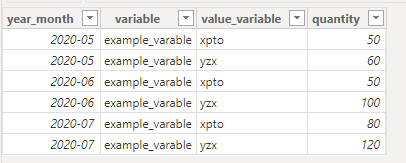cancel
Showing results for
Did you mean:Regular Visitor

## population stability index - DAX

I need to compare a first year month of my distribuition with another year-month distribuition as well , in this example i need to compare 2020-05 with 2020-06,2020-07 and 2020-08.. and so on..

 variable 2020-05 2020-06 2020-07 abc 50% 35% %45 xpto 35% 25% %45 yzx 15% 40% %10

the formula to generate population stability index is is :  Σ([2020-06]-[2020-05])*LN([2020-06]/[2020-05])

I have a table in this format

 year_month variable value_variable quantity 2020-05 example_varable xpto 50 2020-06 example_varable xpto 50 2020-06 example_varable yzx 100

``````PSX =
VAR base_line =
SUMMARIZE (
FILTER ( my_tb, my_tb[yearmonth] = "202005" ),
Sheet1[variable],
Sheet1[value_variable],
Sheet1[yearmonth]
),
"qtd_tp_bl", CALCULATE ( SUM ( Sheet1[qty] ) ),
"total_tp_bl", CALCULATE ( SUM ( Sheet1[qty] ), ( Sheet1[value_variable] ) )
)
VAR base_line_temp =
SELECTCOLUMNS (
base_line,
"qtd_bl", [qtd_tp_bl],
"total_bl", [total_tp_bl],
"Variavel", [variable],
"valor_Variavel", [value_variable]
)
VAR tab_principal =
SUMMARIZE ( Sheet1, Sheet1[var], Sheet1[valor], Sheet1[anomes] ),
"qtd_tp", CALCULATE ( SUM ( Sheet1[qtd] ) ),
"total_tp", CALCULATE ( SUM ( Sheet1[qtd] ), ( Sheet1[valor] ) )
)
VAR tab_principal_temp =
SELECTCOLUMNS (
tab_principal,
"qtd", [qtd_tp],
"total", [total_tp],
"anomes", [yearmonth],
"Variavel", [variable],
"valor_Variavel", [value_variable]
)
VAR uni_mer=
NATURALLEFTOUTERJOIN ( tab_principal_temp, base_line_temp )

VAR tab_calculos =
ADDCOLUMNS ( uni_mer, "Calc", DIVIDE ( [qtd], [total], 0 ) ),
"Base_Line_calc", DIVIDE ( [QTD_BL], [total_bl], 0 )
)
VAR tab_final =
SUMMARIZE ( tab_calculos, [yearmonth], [Calc], [Base_Line_calc] ),
"PS_I",
( [Calc] - [Base_Line_calc] )
* IF (
DIVIDE ( [Calc], [Base_Line_calc], 0 ) = 0,
0,
LN ( DIVIDE ( [Calc], [Base_Line_calc], 0 ) )
)
)
RETURN
SUMX ( tab_final, [PS_I] )``````

in the end , returning zero by monthwhen I generate the table before sumx, worked perfectly

someone knows why this happens in measure? , I guess that is something related with context, but I couldn't find the solution

1 ACCEPTED SOLUTIONCommunity Support

Hi, @thisanco

You can try the following methods.

Sample data:Measure:

``````Previous quantity =
Var _Previous_month=MAXX(FILTER(ALLEXCEPT('Table','Table'[value_variable]),[year_month]<SELECTEDVALUE('Table'[year_month])),[year_month])
Return
CALCULATE(SUM('Table'[quantity]),FILTER(ALLEXCEPT('Table','Table'[value_variable]),[year_month]=_Previous_month))``````
``````Result =
Var _Currentquantity=SUM('Table'[quantity])
return
IF(ISBLANK(_Currentquantity) || ISBLANK([Previous quantity]),BLANK(),
SUMX('Table',_Currentquantity-[Previous quantity])*LN(_Currentquantity/[Previous quantity]))``````Is this the result you expect?

Best Regards,

Community Support Team _Charlotte

If this post helps, then please consider Accept it as the solution to help the other members find it more quickly.Community Support

Hi, @thisanco

You can try the following methods.

Sample data:Measure:

``````Previous quantity =
Var _Previous_month=MAXX(FILTER(ALLEXCEPT('Table','Table'[value_variable]),[year_month]<SELECTEDVALUE('Table'[year_month])),[year_month])
Return
CALCULATE(SUM('Table'[quantity]),FILTER(ALLEXCEPT('Table','Table'[value_variable]),[year_month]=_Previous_month))``````
``````Result =
Var _Currentquantity=SUM('Table'[quantity])
return
IF(ISBLANK(_Currentquantity) || ISBLANK([Previous quantity]),BLANK(),
SUMX('Table',_Currentquantity-[Previous quantity])*LN(_Currentquantity/[Previous quantity]))``````Is this the result you expect?

Best Regards,

Community Support Team _Charlotte

If this post helps, then please consider Accept it as the solution to help the other members find it more quickly.